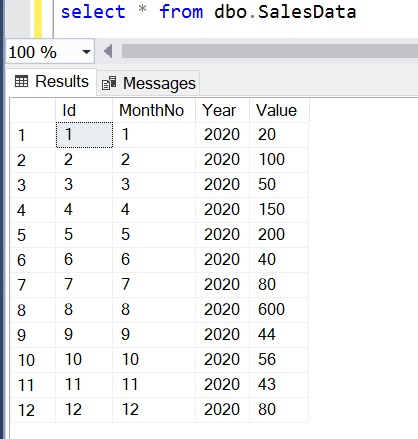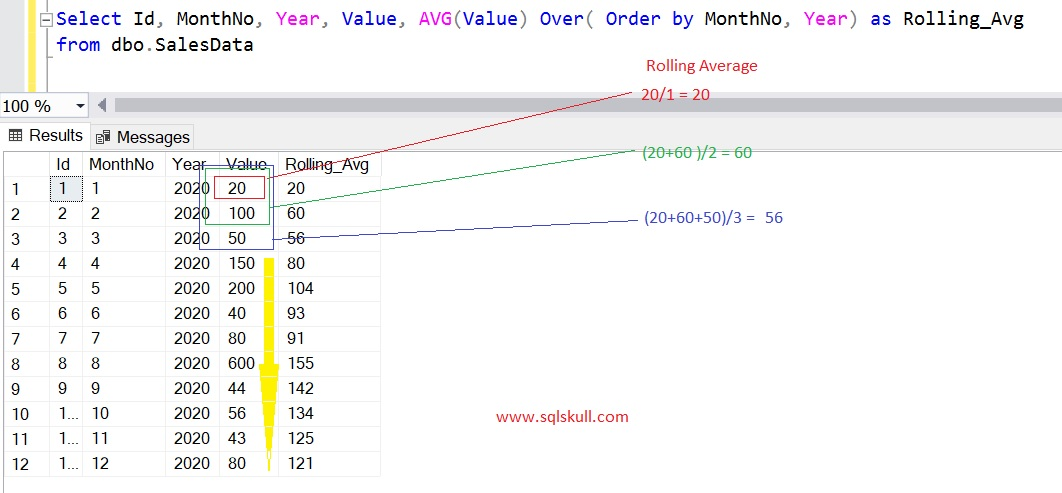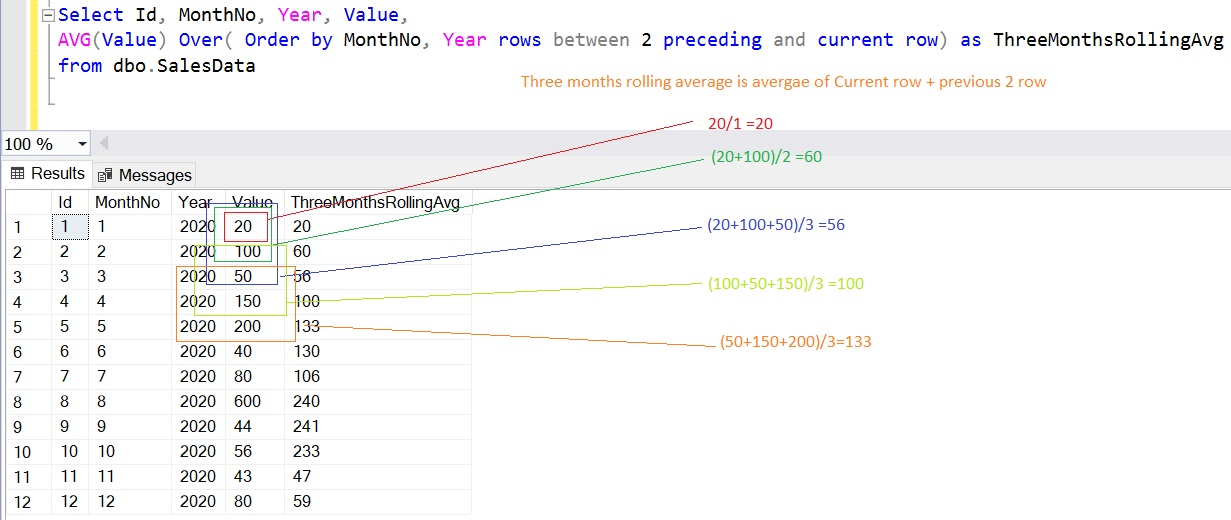Home » Rolling average in SQL

# Rolling average in SQLRolling average is a calculation to analyze data by creating series of average of different subsets of the entire data.

For example, you have data of 12 months and you are asked to find out their average. So when you create the calculation for rolling average. You will get to know, how the average changes as the average rolls or moves for each new month.

Here we have a sample table scripts.

``` Create table dbo.SalesData
(Id int, MonthNo Int, Value Int)

Insert into dbo.SalesData (MonthNo, Year, Value)
values
(1, 2020, 20),
(2, 2020, 100),
(3, 2020, 50),
(4, 2020, 150),
(5, 2020, 200),
(6, 2020, 40),
(7, 2020, 80),
(8, 2020, 600),
(9, 2020, 44),
(10, 2020, 56),
(11, 2020, 43),
(12, 2020, 80)```

Now we have a sample table named SalesData.

`select * from SalesData.`Lets calculate the rolling average, following query returns the rolling average.

```Select Id, MonthNo, Year, Value, AVG(Value) Over( Order by MonthNo, Year) as Rolling_Avg
from dbo.SalesData```

You can see the query output, it returns the rolling average.Lets calculate the last three months rolling average.

Following query returns the last three months rolling average.

```Select Id, MonthNo, Year, Value,
AVG(Value) Over( Order by MonthNo, Year rows between 2 preceding and current row) as ThreeMonthsRollingAvg
from dbo.SalesData```

Lets understand the above query.

Here we have used ROWS clause, the use of the ROWS clause is to limits the rows within a partition by specifying a fixed number of rows preceding or following the current row. The syntax is:

`ROWS BETWEEN lower_bound AND upper_bound`

The bounds can be any of these five options:

• n PRECEDING – It includes n rows before the current row.
• UNBOUNDED PRECEDING – It includes all the rows before the current row.
• CURRENT ROW – It indicates the current row.
• n FOLLOWING – It includes all the n rows after the current row.
• UNBOUNDED FOLLOWING – It includes all the rows after the current row.

So, the query using a window function AVG on a set of values ordered by MonthNo and Year.

The clause ROWS BETWEEN 2 PRECEDING AND CURRENT ROW indicates that the average must be evaluated only using the Value of the current row and the two previous rows.

For each row in the result set the rolling average will be evaluated based on a different set of three Value values.